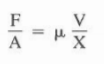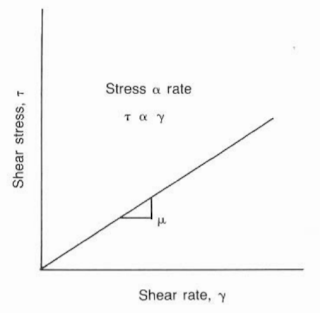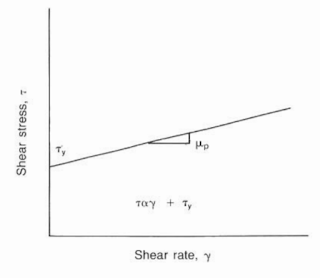## Drilling Mud Flow Regimes

Flow Regimes are the behavior of the fluid while flowing in a well. The most common regimes are laminar, turbulent, and transitional. Unfortunately, it is impossible to clearly define each type in the well. As an example, mud flow may be predominantly laminar, although the flow near the pipe waIls during pipe rotation may be turbulent.

### 1) Laminar Flow.

The most common annular flow regime is laminar. It exists from very low pump rates to the rate at which turbulence begins. Characteristics of laminar flow useful to the drilling engineer are low friction pressures and minimum hole erosion.Laminar Flow Regimes

#### Lamina flow Description

Laminar flow can be described as individual layers, or laminae, moving through the pipe or annulus. The center layers usuaIly move at rates greater than the layers near the weIlbore or pipe. The flow profile describes the variations in layer velocities. These variations are controlled by the shear resistant capabilities of the mud. A high yield point for the mud tends to make the layers
move at more uniform rates.

#### Laminar flow Associated Problems And Solution

Problem
Cuttings removal is more difficult with laminar flow. The cuttings appear to move outward from the higher-velocity layers to the more acquiescent areas. These outer layers have very low velocities and may not be effective in removing the cuttings.

Solution
1. By increasing the yield point, which decreases layer velocity variations.
2. Pumping a 10-20 bbls high-viscosity pill to "sweep"the annulus of cuttings.

### 2) Turbulent Flow.

Turbulence occurs when increased velocities between the layers create shear strengths exceeding the ability of the mud to remain in laminar flow. The layered structure becomes chaotic and turbulentTurbulent Flow Regime
Turbulence occurs commonly in the drill string and occasionally around the drill collars.

#### Turbulent flow Associated Problems

Much published literature suggests that annular turbulent flow increases hole erosion problems. The flow stream is continuously swirling into the walls. In addition, the velocity at the walls is significantly greater than the wall layer in laminar flow. Many industry personnel believe that turbulent flow and the formation type are the controlling parameters for erosion.

### 3) Transitional Flow.

Unfortunately, it is often difficult to estimate the flow rate at which turbulence will occur. In addition, turbulence may occur in various stages. It is convenient to describe this "gray" area as a transitional stage.

### Flow Regimes Turbulence Criteria.

Several common methods can be used to establish turbulence criteria. The most common approach is the Reynolds number. Others include:
1) Intersection of the flow rate vs pressure loss calculations for laminar and turbulent flow.
2) Calculation of a z-value.

Reynolds published a paper (1883) that reported experiments dealing with flow in pipes. He injected a thin filament of dye into a moving stream of liquid flowing in a glass pipe and found that if the numerical value of a group of variables was less than 2,100, the dye filament remained small. The
filament rapidly dispersed in "eddies" if the value of the group was greater than 2,100.

Turbulence occurs when the ratio of the momentum of the liquid to the viscosity ability of the liquid to dampen permeations exceeds some empirically determined value. The momentum force of the liquid is its velocity times its density. The viscous ability of the liquid to damp out permeations is the internal resistance against change and the effects of the walls of the borehole.

#### Newtonian Fluids

For the simple case of a Newtonian, nonelastic liquid flowing in a pipe, the dampening effect is the quotient of the viscosity and the diameter of the wellbore:

Where:
NR = Reynolds number
ρ = density
= diameter
μ = viscosity

#### Non-Newtonian Fluids

Since drilling muds are non-Newtonian and contain some degree of elasticity, definition of a single Reynolds number becomes difficult.

The Reynolds number for flow of a non-Newtonian liquid in a pipe will be as following:

Where:
NRc = effective Reynolds number
n = flow behavior index
= consistency index

The terms n and K relate to the Power Law mud model, which will be discussed in following sections.
• A simpler equation used in the literature to predict the Reynolds number at the upper limit of laminar flow is as follows:
NRc= 3,470 - 1,370 n

• The relation for the Reynold~ number between the transition and turbulent flow regimes :
NRc= 4,270 - 1,370 n

It is obvious from above Eqs. that the Reynolds number is sliding, with its dependency on the flow behavior index.
The position of intersection between the laminar and turbulent flow pressure losses depends on the equations being used see below figure., The Reed slide rule or. the Hughes tables can give errors if the mud is quite non-Newtonian at the applicable shear rate. Using well-known equations available in the literature and a Newtonian fluid, the laminar-turbulent pressure lines intersect at a point that
is equivalent to a Reynolds number of approximately 1,900, or 10% below the 2,100 value. The point of intersection for non-Newtonian fluids may be 30% below the actual transition.

####Intersection of laminar and turbulence flow calculations used as the turbulence criteria

The z-value method was developed by Ryan and Johnson based on experimental data from several sources and experimentally verified by others. The z-value is calculated from below Eq.:

Laminar flow is assumed, and the z-value is calculated and plotted vs the radius. If the value exceeds 808, the assumption of laminar flow'is incorrect and turbulence occurs. Calculating the z-value is complex and time-consuming

### Flow Regimes Critical Velocity.

The term critical velocity is used to define the sit--ngle velocity at which the flow regime changes from laminar to turbulent. This variable from above Eq. is the most important since all other members are considered constant in a typical operation. Since no single Reynolds number defines the transitional zone, it follows that a range of critical velocities may be necessary to determine the flow regime.

In practical applications, a critical velocity (Vc) and an actual velocity (Va) are calculated.
• If Va < Vc, the flow is laminar.
• If Vc < Va, the flow is turbulent.
• If Va = Vc, calculations are made with both flow regimes and the larger pressure losses are used.

## Drilling Mud Flow Regimes (Mathematical) Models

A mathematical model is used to:
1. Describe the fluid behavior under dynamic conditions.
2. Calculate friction pressures
3. Calculate swab and surge pressures
4. Calculate slip velocities of cuttings in fluids.
The models most commonly used in the drilling industry are:
1. Newtonian Model.
2. Bingham Plastic Model.
3. Power Law Model.

### Shear Stress & Shear Rate

Terms used in mud models are shear stress and shear rate. They can be described by considering two plates separated by a specified distance with a fluid. If a force is applied to the upper plate while the lower plate is stationary, a velocity will be reached and will be a function of the force, the distance between the plates, the area of exposure, and the fluid viscosity:Relation Between shear Stress and Shear Rate
Where:
F = force applied to the plate
A = contact area
V = plate velocity
X = plate spacing
μ = fluid viscosity
F/A is termed the shear stress (𝜏)
V/X is shear rate (ɣ):

### Newtonian Model

In drilling operations, the shear stress and shear rate are analogous to pump pressure and rate, respectively. Newtonian Fluids. The model used initially to describe drilling mud was the Newtonian model.
It stated that pump pressure (shear stress) would increase proportionally to shear rate as seen in below figure. If a constant of proportionality is applied to represent fluid viscosity, above equation will become as following:

Where:
μ = fluid viscosityNewtonian Model

Unfortunately, drilling muds usually cannot be described by a single viscosity term. They require two or more points for an accurate representation of behavior. As a result, the Newtonian model generally is not used in hydraulics plans.

### Bingham Plastic Model

The Bingham model was developed to describe more effectively drilling muds presently in use. Bingham theorized that some amount of stress would be required to overcome the mud's gel structure before it would initiate movement as per below figure:Bingham Model
which can be described by this equation:
In practical terms, the equation states that a certain pressure would be applied to the mud to initiate movement. Flowing mud pressures would be a function of the initial yield pressure and the fluid viscosity. Shear rates are normally taken at 300- and 600-rpm rates on the viscometer.

The fluid viscosity (μp)and the yield stress (𝜏y)are calculated as follows:

#### Plastic Viscosity

The fluid viscosity then will be termed plastic viscosity (PV) due to the plastic nature of the fluid and is measured in centipoise (cp). The plastic viscosity is affected by the size, shape, and concentration of particles in the mud system. As mud solids increase, the plastic viscosity increases. The plastic viscosity is a mud property that is not affected by most chemical thinners and can be controlled only by altering the state or number of solids.

#### Yield Stress

The yield stress, 𝜏y, is given the name of yield point and is measured in Ib/100 ft^2. It is a function of the interparticle attraction of the solids in the mud. The yield point is controlled by chemical thinners, dispersants, and viscosifiers.

#### Common Mud Properties

Below tables illustrate common mud properties for gel-based, Oil Based Mud, and invert oil emulsions. These properties were obtained from various mud companies and should be used only as a guide.

In fact, it is difficult to justify the use of PV and YP terms to oil muds due to the manner in which emulsified wateris treatedas a solidparticle. . Oil Based Mud Figure can be used to describe the weaknesses of the Bingham model. A problem with any model used in drilling operations is its dependence on using two points to define a line that should be known, intuitively, to be nonlinear.

The 300- and 600-rpm shear rates are generally greater than annulus shear rates, resulting in calculated shear stresses greater than the actual values. Although the Bingham model is commonly accepted in practice, a model such as the Power Law would be more descriptive, particularly when the fluid is in turbulence.

### Power Law Model

The Power Law model is a standard mathematical expression used to describe a nonlinear curve. The equation for drilling fluids is shown in the below Eq.

Where:
K = consistency index
n = flow behavior index
The flow behavior index is descriptive of the degree to which the fluid is non-Newtonian.
The flow behavior and consistency indices are calculated from below Eqs., respectively (is modified slightly for use in Moore's slip velocity correlation. will be discussed later):

(Above Eq. is modified slightly for use in Moore's slip velocity correlation. will be discussed later)

This Equation can be described as in below figurePower Law Model

### Example For using Power Low Model & Bingham Model:

Use the following viscometer readings to compute PV, YP, n, and K:

θ600 = 64
θ300 = 35

Solution:
1. PV = θ600 - θ300 = 64-35 = 29 cp
2. YP = θ300 - PV = 35-29 = 6 Ib / 100 ft^2
3. n = 3.32 x Log ( θ600 / θ300 ) = 3.32 x Log ( 64 / 35) = 0.870
4. K = θ300 / 511^ n = 35 / 511 ^ 0.870 = 0.154